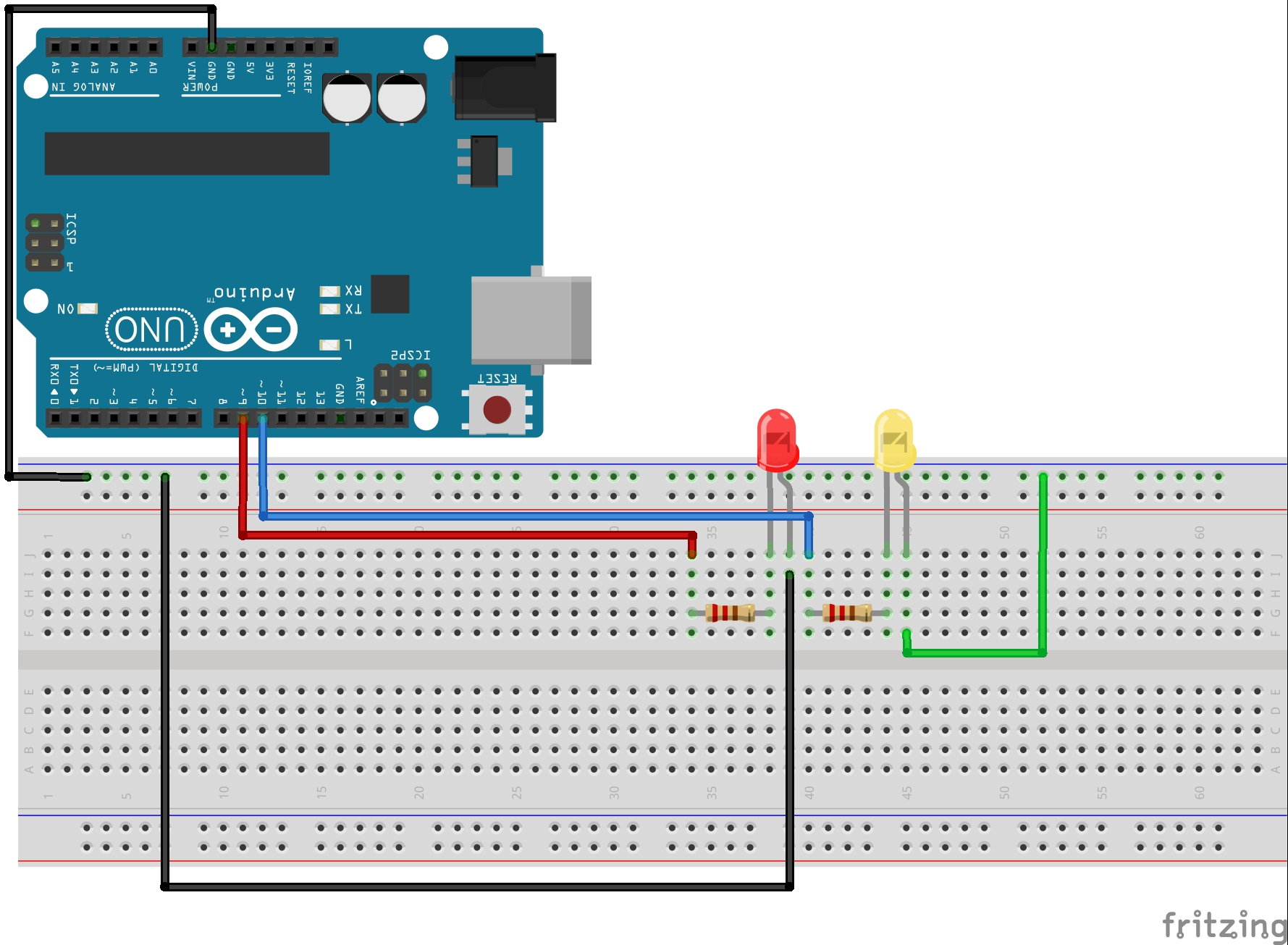# Lesson 8: Writing Analog Voltages in Arduino

We have done some pretty cool stuff so far with the arduino. We have learned how to get input from the user, and how to send information to the user. We have learned how to control commands with both for loops and while loops. We are well on our way to building some really powerful projects. The thing is, so far all of our commands to the arduino pins have in effect been to either turn the pin On or turn it Off. That is, when we digitalWrite HIGH or LOW to the pins, we are either turning on the full 5 volts or turning it all the way off. The truth is that most times we want something in the middle. We would maybe want a voltage of 2.3 volts.

The arduino pins with the squiggly line by them are able to write these in between voltages. These are pins 3,5,6,9,10,11 on the arduino uno.

In the world of engineering and electronics, we say that we want an analog voltage. That is, we want to apply any voltage we want, not just 0 or 5. To output an arbitrary voltage between 0 and 5, would issue the arduino an analogWrite command. Unfortunately, the arguments for the analogWrite command are not as simple as telling it a number between 0 and 5. We must give it an integer between 0 and 255. If we issued the command analogWrite(mypin,0), it would apply 0 volts to mypin. If we issued the command analogWrite(mypin,255), it would appy 5 volts to the pin. As you can see, if we gave the command analogWrite(mypin, 127), we would get about 2.5 volts applied to mypin. You can see those are the easy ones, but in order to figure out exactly what value we should use for exactly the voltage we want, we will need to do some math. Remember all the times you had to calculate the equation of a line in math? Well you are going to do it for real now and for a reason. We need to get an equation that will allow us to calculate the Write Value we should use to get the Voltage

You can see that you need the equation for the line above. Good news! You have two points so can calculate the equation of the line. The first point is (0,0), that is to say, that if you want a voltage of 0 to be applied to the pin, you should analogWrite the value of 0, as we explained above. The second point is (5,255), that is to say, if you want to apply a voltage of 5 Volts, you should analogWrite the value 255, as explained above. Now we calculate the equation of the line. the slope would be:

m = (y2-y1)/(x2-x1) = (255-0)/(5-0) = 51

OK, now to get the equation of the line we will use the point slope form of a line, and we get:

(y-y1)=m(x-x1)

We can plug in the point (0,0) and get

(y-0) = m(x – 0)

And when we plug in 51 for m we get:

y = 51X

Remember X is the voltage we want, and Y is the value we write, so this equation can be rewritten:

Write Value = 51 X (Desired Voltage)

So, this equation lets us calculate precisely what value we should analogWrite in order to get the voltage we want on the pin. I hope this makes sense. If you are confused watch the video and it will make more sense.

The bottom line is that we can use this equation to calculate the number we should write to get the voltage that we want at a pin.

As an example, if we wanted to get exactly 2 volts, we should write the value 2X51= 102. If we wanted two volts on the pin myPin, we would issue the command analogWrite(mypin, 102).

Now lets start playing around with a circuit. Lets use the circuit we have been using the last few lessons. Hopefully you still have it hooked up, but if you need help we take you through it step-by-step in Lesson 3.This circuit will allow you to independently control two Light Emitting Diodes from the arduino microcontroller